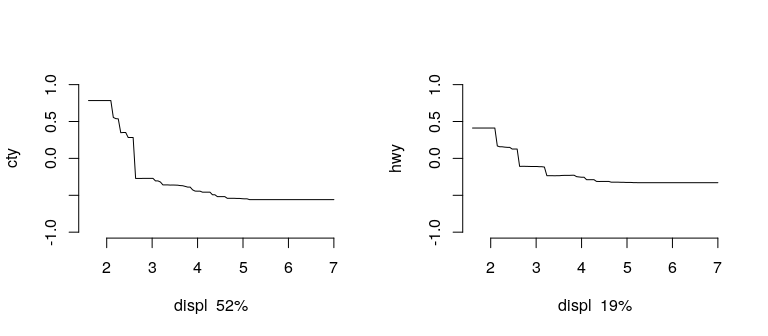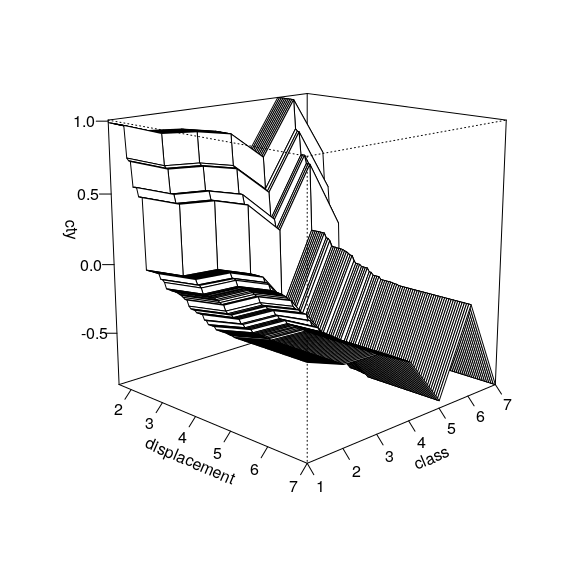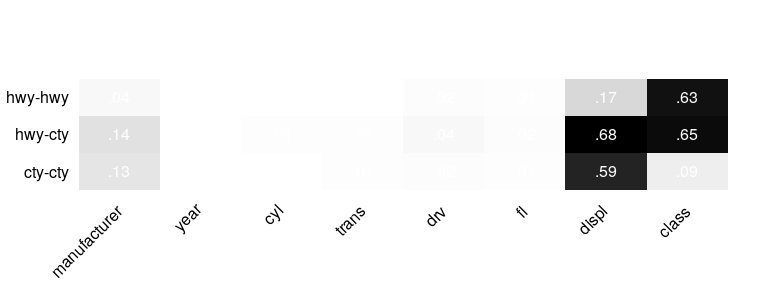# mvtboost example 1: mpg

#### 2016-12-05

Boosted decision tree ensembles (Friedman, 2001) are a powerful off-the-shelf learning algorithm, allowing dependent variables to be non-linear functions of predictors as well as handling predictors with missing data. In addition to having high prediction performance, Boosted decision trees are an extremely flexible approach for exploratory data analysis.

A well known R package for fitting boosted decision trees is gbm. This package extends gbm to multivariate, continuous outcome variables by fitting a separate univariate model of a common set of predictors to each outcome variable. This common basis accounts for covariance in the outcome variables as in seemingly unrelated regression. We refer to the package gbm and the extensive literature on boosting with decision trees for theoretical and technical details about how such a model is fit and interpreted (see references below).

While it is in principle not too complex to fit separate tree models to each outcome variable, considering the outcome variables jointly has several benefits which the package makes possible:

1. The number of trees and shrinkage can be chosen to jointly minimize prediction error in a test set (or cross validation error) over all outcomes.
2. It very easy to compare tree models across outcomes.
3. The ‘covariance explained’ by predictors in pairs of outcomes can be estimated

In general, the joint analysis of several outcome variables can be informative. We illustrate the use of multivariate tree boosting by exploring the mpg data from ggplot2, investigating features of cars that explain both city and highway fuel efficiency (mpg).

## 1. Fitting the model

Fitting the model is very similar to gbm.fit. Standardizing the outcomes is recommended.

library(mvtboost)
data("mpg",package="ggplot2")
Y <- mpg[,c("cty","hwy")]      # use both city and highway mileage as dvs
Ys <- scale(Y)                 # recommended that outcomes are on same scale
X <- mpg[,-c(2,8:9)]           # manufacturer, displacement, year, cylinder, transmission,drive, class
char.ids <- unlist(lapply(X,is.character))
X[,char.ids] <- lapply(X[,char.ids],as.factor)

out <- mvtb(Y=Ys,X=X,          # data
n.trees=1000,          # number of trees
shrinkage=.01,         # shrinkage or learning rate
interaction.depth=3)   # tree or interaction depth

### 1.1 Tuning the model

The model can be tuned using either (or both) a test set or cross-validation. Cross-validation can be easily parallelized by specifying mc.cores. Here bag.fraction is set to .5, so that each tree is fit to a different random sub-sample of half the data.

out2 <- mvtb(Y=Ys,X=X,
n.trees=1000,
shrinkage=.01,
interaction.depth=3,

bag.fraction=.5,      # fit each tree to a sub sample of this fraction
train.fraction=.5,    # only fit the model to this fraction of the data set
cv.folds=3,           # number of cross-validation folds
mc.cores=1,           # run the cross-validation in parallel
seednum=103)          # set the seed number for reproducibility
out2$best.trees ##$best.testerr
##  998
##
## $best.cv ##  670 ## ##$last
##  1000

## 2. Interpreting the model

The summary of the fitted model shows the best number of trees (the minimum of training, test or CV error if available), the relative influences of each predictor for each outcome.

After tuning with cross-validation, results change slightly.

summary(out)
## $best.trees ##  1000 ## ##$relative.influence
##                cty   hwy
## manufacturer  8.33  4.09
## displ        60.21 20.15
## year          0.74  1.53
## cyl           8.75  5.04
## trans         2.03  0.60
## drv           1.02 10.96
## fl            3.62  2.85
## class        15.30 54.78
summary(out2)
## $best.trees ##  670 ## ##$relative.influence
##                cty   hwy
## manufacturer 23.93 15.10
## displ        52.01 18.76
## year          0.39  0.36
## cyl           2.71  3.60
## trans         4.37  4.27
## drv           1.77  1.85
## fl            2.45  1.77
## class        12.37 54.29

### 2.1 Predictions

The predicted values of the model can be easily computed using the standard predict function. The variance explained ($$R^2$$) is shown below. By default, the number of trees used is the minimum of the best trees given by CV, test, or training error.

yhat <- predict(out2,newdata=X)
(r2 <- var(yhat)/var(Ys))
##           cty       hwy
## cty 0.7088217 0.7276086
## hwy 0.7276086 0.7459731

### 2.2 Univariate and Partial Dependence Plots

Univariate and multivariate partial-dependence plots can highlight non-linear effects of predictors (Friedman, 2001). Below, we show the effect of displacement on city and highway miles per gallon. Because mpg has been standardized, increases in $$x$$ correspond to standard deviation changes in either city or highway mpg. We see that displacement has a larger effect on city mpg than highway mpg.

par(mfcol=c(1,2))              # model implied effects for predictor 2 for cty and hwy
plot(out2,response.no=1,predictor.no=2,ylim=c(-1,1))
plot(out2,response.no=2,predictor.no=2,ylim=c(-1,1))We can also obtain the model implied effects as a function of two predictors:

mvtb.perspec(out2,response.no = 1,predictor.no = c(2,8),xlab="displacement",ylab="class",theta=45,zlab="cty")### 2.3 Detecting departures from additivity

Decision tree ensembles can approximate multi-way interactions, but multi-way interactions are difficult to detect. The function mvtb.nonlin detects when the model implied predictions depart from additivity as a function of all pairs of predictors. This heuristic may indicate the presence of non-linear and interaction effects.

Below, we show an example of computing departures from additivity. Pairs of predictors with significant non-linear effects might be plotted (as above) to investigate whether 2-way interactions exist. Below, we show that the most important non-linear effects all involve displacement, which has a very large non-linear effect.

nonlin.out <- mvtb.nonlin(out2,X=X,Y=Y)
nonlin.out$hwy$rank.list
##   var1.index var1.names var2.index var2.names nonlin.size
## 1          4        cyl          2      displ    18.54248
## 2          3       year          2      displ    18.52665
## 3          7         fl          2      displ    18.40515
nonlin.out$cty$rank.list
##   var1.index var1.names var2.index   var2.names nonlin.size
## 1          2      displ          1 manufacturer    73.27942
## 2          7         fl          2        displ    69.70730
## 3          3       year          2        displ    69.37871

### 2.4 Covariance explained

One of the important features of considering multivariate outcomes jointly is the possibility of estimating the covariance between pairs of outcome variables as functions of individual predictors. This is estimated below. The resulting table has rows corresponding to pairs of outcomes, and columns for each predictor. Details are in Miller & Lubke et al., (2016).

covex <- mvtb.covex(out2, Y=Ys, X=X)
round(covex,2)
##         manufacturer displ year  cyl trans  drv   fl class
## cty-cty         0.13  0.59    0 0.01  0.01 0.02 0.01  0.09
## hwy-cty         0.14  0.68    0 0.01  0.01 0.04 0.02  0.65
## hwy-hwy         0.04  0.17    0 0.00  0.00 0.02 0.01  0.63

### Clustering the covariance explained matrix

If the number of predictors/outcomes is large, interpreting the matrix is challenging. The covariance explained matrix can be clustered by grouping the predictors that explain covariance in similar pairs of outcomes. This is done by hierarchical clustering of the distance between columns (predictors) and the rows (pairs of outcomes).

Below, we cluster the covariance explained matrix, and display it as a heat map. Note that the method of computing the distance between covariance matrices dist.method and method of clustering the rows and columns clust.method can be changed to result in different clustering solutions.

cc <- mvtb.cluster(covex, clust.method = "ward.D", dist.method = "manhattan")
round(cc,2)
##         manufacturer year  cyl trans  drv   fl displ class
## cty-cty         0.13    0 0.01  0.01 0.02 0.01  0.59  0.09
## hwy-cty         0.14    0 0.01  0.01 0.04 0.02  0.68  0.65
## hwy-hwy         0.04    0 0.00  0.00 0.02 0.01  0.17  0.63
mvtb.heat(covex)